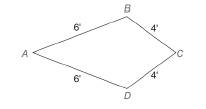Chapter 4.2, Problem 6EElementary Geometry For College St...

7th Edition
Alexander + 2 others
ISBN: 9781337614085

Solutions

Chapter
SectionElementary Geometry For College St...

7th Edition
Alexander + 2 others
ISBN: 9781337614085
Textbook Problem

A carpenter joins four boards of lengths 6 ft, 6 ft, 4 ft, and 4 ft, in that order, to form quadrilateral A B C D as shown.a) What type of quadrilateral is formed?b) How are angles B and D related?To determine

a)

The type of the quadrilateral formed.

Explanation

Given,

The lengths of the boards are 6 ft, 6 ft, 4 ft and 4 ft.

Here,

AB=6 ft

BC=4 ft

CD=4 ft, and

DA=6 ft.

In the given figure the pairs of the adjacent sides are congruent

To determine

b)

The relation by which the angles B and D related.

Still sussing out bartleby?

Check out a sample textbook solution.

See a sample solution

The Solution to Your Study Problems

Bartleby provides explanations to thousands of textbook problems written by our experts, many with advanced degrees!

Get Started

Find more solutions based on key concepts